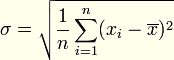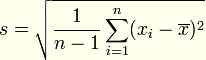##### Page tree
Go to start of banner

# %SampleStandardDeviation function

Function
The function calculates the sample standard deviation from the valid values in the column of object Structured variable.
Declaration
```REAL %SampleStandardDeviation(
in StructVar^Column
)```

Parameters
 StructVar Object of Structured variable type. Column Column of structured variable.

Description
The standard deviation is defined as a positive square root of variance. It is calculated if there is available complete set of possible states of the process (system).The sample standard deviation is defined as the square root of sample variance. It is calculated from the selection, i.e. if there is not available complete set of possible states but just the selection of them. Therefore, there must be used n-1 (because the one measuring is dependent, with the calculation of mean value). For a large number of measurements, the difference between standard deviation and the sample standard deviation decreases.Example
`%SampleStandardDeviation(SV.Vektor^Values) `

Related pages:

Write a comment…